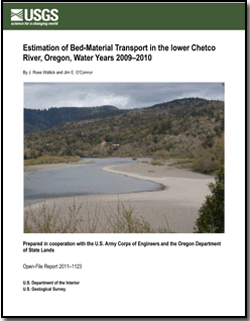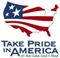Open-File Report 2011–1123

## Estimation of Bed-Material Transport in the Lower Chetco River, Oregon, Water Years 2009–2010

### SIGNIFICANT FINDINGSThis assessment of bed-material transport uses methods developed in a previous study (Wallick and others, 2010) to estimate bed-material flux at the USGS Chetco River streamflow gaging station located at flood-plain kilometer 15 (14400000). On the basis of regressions between daily mean flow and transport capacity, daily bed-material flux was calculated for the period October 1, 2008 to March 30, 2011. The daily flux estimates were then aggregated by water year (WY) for WY 2009 and WY 2010 and the period April 1–March 31 during 2008–09, 2009–10 and 2010–11. The main findings were:

•   Estimated bed-material flux for WY 2009 (October 1, 2008 to September 30, 2009) was 87,300 metric tons as calculated by the Parker (1990a, b) equation (hereinafter "the Parker equation") and 116,900 metric tons as calculated by the Wilcock and Crowe (2003) equation (hereinafter "the Wilcock–Crowe equation").

•   Estimated bed-material flux for water year 2010 (October 1, 2008 to September 30, 2009) was 56,800 metric tons as calculated by the Parker equation and 96,700 metric tons as calculated by the Wilcock–Crowe equation.

•   Estimated bed-material flux for April 1, 2008, to March 31, 2009, was 84,700 metric tons as calculated by the Parker equation and 111,700 metric tons as calculated by the Wilcock–Crowe equation. Flux values from April 1 to September 30, 2008, are from Wallick and others (2010).

•   Estimated bed-material flux for April 1, 2009, to March 31, 2010, was 45,500 metric tons as calculated by the Parker equation and 79,100 metric tons as calculated by the Wilcock–Crowe equation.

•   Estimated bed-material flux for April 1, 2010, to March 31, 2011, was 67,100 metric tons as calculated by the Parker equation and 134,300 metric tons as calculated by the Wilcock–Crowe equation. These calculations used provisional flow data for December 29, 2010, to March 31, 2011, and may be subject to revision.

•   Water years 2009 and 2010 both had less bed-material transport than the average annual transport values of 105,300 and 152,300 metric tons for the period 1970–2010 as calculated by the Parker and Wilcock–Crowe equations, respectively.

First posted May 20, 2011

Director, Oregon Water Science Center
U.S. Geological Survey
2130 SW 5th Avenue
Portland, Oregon 97201
http://or.water.usgs.gov/

### Suggested citation:

Wallick, J.R., and O'Connor, J.E., 2011, Estimation of bed-material transport in the lower Chetco River, Oregon, water years 2009–2010: U.S. Geological Survey Open-File Report 2011–1123, 12 p.

### Contents

Significant Findings

Introduction

Chetco River Study Area

Approach and Key Findings

References CitedU.S. Department of the Interior | U.S. Geological Survey
[an error occurred while processing this directive] URL: https://pubs.usgs.gov
Page Contact Information: USGS Publications Team